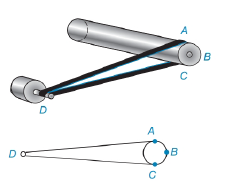Chapter 6.3, Problem 34EElementary Geometry For College St...

7th Edition
Alexander + 2 others
ISBN: 9781337614085

Solutions

Chapter
SectionElementary Geometry For College St...

7th Edition
Alexander + 2 others
ISBN: 9781337614085
Textbook Problem

The drive mechanism on a treadmill is powered by an electric motor. In the figure, find m ∠ D if m A B C ^ is 36 ∘ larger than m A C ^ .To determine

To find:

To find mD

Explanation

Given that, if mABC^ is 36 larger than mAC^.

That is mABC^=mAC^+36°.

From the diagrammatic representation,

Let D be the vertex outside the circle therefore,

mD=mABC^-mAC^2

Since mABC^=mAC^+36°

Substitute m</

Still sussing out bartleby?

Check out a sample textbook solution.

See a sample solution

The Solution to Your Study Problems

Bartleby provides explanations to thousands of textbook problems written by our experts, many with advanced degrees!

Get Started

Evaluate the integral, if it exists. x1x4dx

Single Variable Calculus: Early Transcendentals, Volume I

Explain the difference between a matched-subjects design and a repeated-measures design.

Essentials of Statistics for The Behavioral Sciences (MindTap Course List)

Evaluate the integral. 6. 01x(2x+1)3dx

Single Variable Calculus: Early Transcendentals